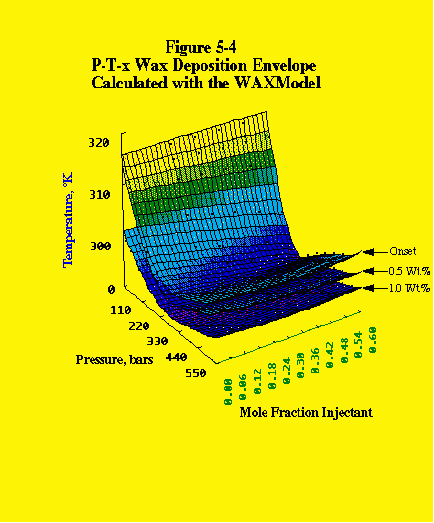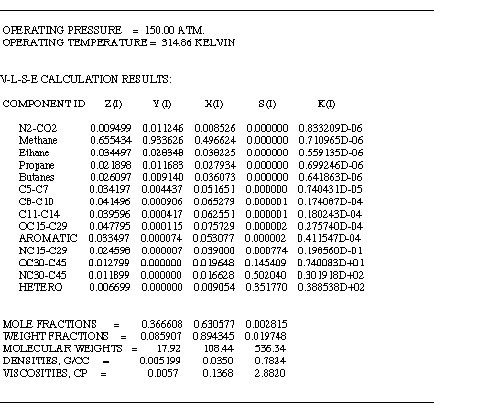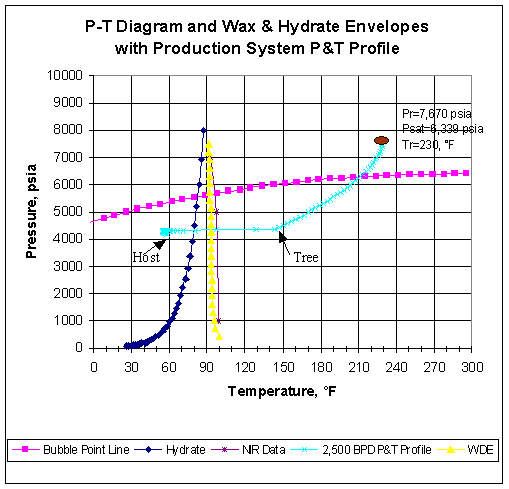WAXModelR by AsphWax

Wax Phase Behavior Simulator

The Thermodynamic Model of Waxes (WAXModelR) computer program is capable of simulating the wax phase behavior of reservoir fluids. The WAXModelR can calculate the complete wax deposition envelope (WDE) of a reservoir fluid, including wax quality lines and much more.

An example calculation of the complete three-dimensional WDE envelope of a reservoir fluid mixture is shown below.An example output of the WAXModelR for a FLASH calculation inside the deposition envelope and below the bubble point line is shown below.An example output of the WAXModelR for a complete P-T Diagram and wax deposition envelope  calculation is shown below.